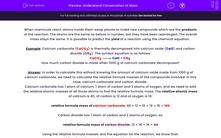# Understand Conservation of Mass

In this worksheet, students will consider the law of conservation of mass and use this to calculate the mass of products in different chemical reactions.Key stage:  KS 4

Year:  GCSE

GCSE Boards:   AQA, AQA Trilogy, AQA Synergy, OCR 21st Century, OCR Gateway, Pearson Edexcel, Eduqas,

Difficulty level:#### Worksheet Overview

When chemicals react, atoms inside them swap places to make new compounds which are the products of the reaction. The atoms are the same as before in number, but they have been rearranged. The overall mass stays the same. It is possible to predict the yield of a reaction using the chemical equation.

Example: Calcium carbonate (CaCO3) is thermally decomposed into calcium oxide (CaO) and carbon dioxide (CO2). The symbol equation is as follows:

CaCO3CaO + CO2

How much carbon dioxide is made when 1000 g of calcium carbonate decomposes?

Answer: In order to calculate this without knowing the amount of calcium oxide made from 1000 g of calcium carbonate, we need to calculate the relative formula masses of the compounds involved. In this case, calcium carbonate and carbon dioxide.

Calcium carbonate has 1 atom of calcium, 1 atom of carbon and 3 atoms of oxygen, and we need to add the relative atomic masses of all those atoms to find the relative formula mass. The relative atomic mass of calcium is 40, of carbon is 12 and of oxygen is 16:

relative formula mass of calcium carbonate: 40 + 12 + 16 + 16 + 16 = 100

Carbon dioxide has 1 atom of carbon and 2 atoms of oxygen, so:

relative formula mass of carbon dioxide: 12 + 16 + 16 = 44

Using the relative formula masses and the equation for the reaction, we know that:

100 g of calcium carbonate gives 44 g of carbon dioxide

1000 g is 10 times more than 100 g, so 1000 g of calcium carbonate gives 10 x 44 = 440 g of carbon dioxide.

Make sure you're confident with how that answer was obtained and then move on to have a go at some questions.

### What is EdPlace?

We're your National Curriculum aligned online education content provider helping each child succeed in English, maths and science from year 1 to GCSE. With an EdPlace account you’ll be able to track and measure progress, helping each child achieve their best. We build confidence and attainment by personalising each child’s learning at a level that suits them.

Get started••••